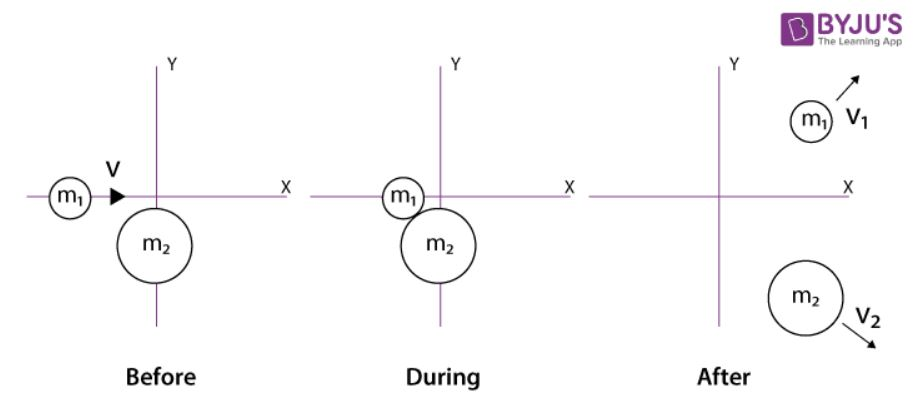Win up to 100% scholarship on Aakash BYJU'S JEE/NEET courses with ABNAT Win up to 100% scholarship on Aakash BYJU'S JEE/NEET courses with ABNAT

# Collision of Point Mass

Much of our knowledge of atoms and nuclei and all of our knowledge of elementary particles is derived from observations of carefully arranged collisions. The basic sequence of a collision is shown in the figure given below, along with a convenient set of reference axes. We will further understand the topic by conducting an experiment.

## Objectives and Aim of the Experiment

To examine some collisions between macroscopic objects quantitatively.

The goal of a collision experiment is to find out how the particles interact during the collision by measuring the masses and velocities of the particles before and after the collision. In the case of microscopic objects, we often do not have direct access to what the particles do during the collision because the lengths and times involved are too small for observation, so there is no more direct way to obtain this information. In this experiment, you will study several simple collisions between macroscopic objects to gain some understanding of how these experiments work and the effects of various conservation laws.

In many cases, conservation laws restrict but do not totally determine the results of a collision. For example, if the force between m1 and m2 is the only force which can change their motion, then both linear and angular momentum will be conserved in the system consisting of m1.

## Example of Collision of Point MassesA collision sequence showing initial and final velocities of m1 and m2.

In the situation shown in the image above, where only the m1 is moving before the collision, this tells us immediately that at least one of the masses must be moving after the collision to carry off the initial momentum.

Conservation of Linear Momentum

More generally, we can express the conservation of linear momentum by the vector relationship,

$$\begin{array}{l}\vec{P_{1}i}+\vec{P_{2}i}=\vec{P_{1}f}+\vec{P_{2}f}…..(1)\end{array}$$

which asserts that all components of linear momentum must be conserved, regardless of what happens during the actual collision. We will work on an air table which constrains the motion to the x-y plane and reduces the frictional forces in that plane nearly to zero, so we would expect Eq. 1 to hold for our examples.

Conservation of Angular Momentum

Angular momentum is a little more complicated because there are two parts to a rigid body. The part due to translational motion is

$$\begin{array}{l}\vec{L_{t}}=\vec{r} \times \vec{mv}…..(2)\end{array}$$

where r is a vector from the chosen, fixed origin to the centre of mass, and v is the velocity of the centre of mass. There is also angular momentum due to the rotation or ‘spin’ of the object about the centre of mass, given by

$$\begin{array}{l}\vec{L_{s}}=I\vec{w}…..(3)\end{array}$$

where I is the moment of inertia about the centre of mass and

$$\begin{array}{l}\vec{\omega }\ \text{is the angular velocity about the centre of mass.}\end{array}$$
The total angular momentum is then L = Ls + Lt, and we can write the conservation of angular momentum in the form

$$\begin{array}{l}\vec{L_{1i}}+\vec{L_{2i}}=\vec{L_{1f}}+\vec{L_{2f}}…..(4)\end{array}$$

If the motion is limited to two dimensions, the translational angular momentum is necessarily perpendicular to the plane of motion. On an air table, the sliders can only rotate about the vertical direction, so the spin angular momentum is also perpendicular to the plane of motion, and Eq. 4 reduces to a single scalar equation. Since only internal forces act in the plane of motion, there are no external torques to change the angular momentum of the system, and we expect Eq. 4 to hold for the collisions we will study.

Kinetic Energy

Although unlikely for macroscopic objects, it may also happen that the kinetic energy remains constant during the collision so that

$$\begin{array}{l}E_{1i}+E_{2i}=E_{1f}+E_{2f}…..(5)\end{array}$$

As with the angular momentum, the kinetic energy of a rigid object can be thought of as the sum of a piece due to the translation of the centre of mass and a piece due to rotation about the centre of mass.

$$\begin{array}{l}E=\frac{1}{2}\;mv^{2}\;+\;\frac{1}{2}\;Iw^{2}…..(6)\end{array}$$

If Eq. 5 is obeyed, the collision is said to be elastic, otherwise, it is inelastic. Some people restrict the term elastic to situations where the translational energy alone is constant. Since we do not know much about what happens when two objects hit each other, we will have to discover experimentally whether our collisions are elastic or inelastic. For a collision in two dimensions with known starting conditions, there are four unknown linear velocity components and two angular speeds after the collision. Eqs. 1, 4 and 5 supply at most four restrictions on these six quantities, and in fact, only three if the collision is not known to be elastic.

The final velocities must, therefore, be determined by the details of the forces acting during the collision. By measuring the final velocities for various initial conditions, one can learn about the interaction force between the particles, at least in principle. During the course of the experiment, you will see qualitatively how this works and also see how the conservation laws are applied to a real collision.

### Collision with Reduced Mass – Video Lesson## Frequently Asked Questions on Collision of Point Mass

Q1

### After a collision, how do you determine the mass?

The total momentum is simply the total mass of the colliding objects multiplied by their velocity because they all travel in the same direction after the impact.

Q2

### What happens after the collision if the masses of the two objects are different?

According to Newton’s second law of motion, the acceleration of an object is determined by both force and mass. If the colliding objects have uneven masses, the contact force generated during the impact will cause unequal accelerations.

Q3

### In an elastic collision, what happens to the masses?

When a small mass approaches a larger mass, a collision happens. Whenever a larger mass approaches a smaller mass, both masses retain momentum in the same direction.

Test Your Knowledge on Collision Of Point Mass